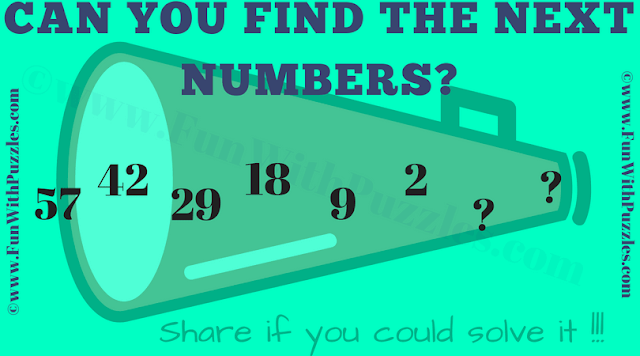This Math Series Picture Riddle is for school going students. In this Math Series Riddle, you are given one number series. The Numbers given in this series follows some Mathematical Sequence or Pattern. Can you decipher this pattern which makes this Math Series and find the next numbers in this series?Can you find the next numbers in this Math Series?